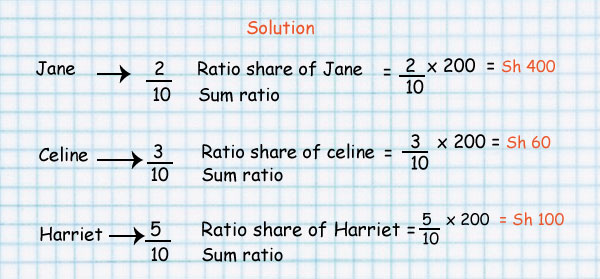Comparing using ratio

#### Example 1

Simplify the following ratios:

200 cm to 3m

#### Solution

Make the units to be the same.

1 m = 100 cm then,

3 m= 3 X 100 = 300 cm

= 200 cm to 300 cm

=200/300

= 2:3

#### Example 2

Juma walked 100 m, Hajj walked 2 km and Ali walked 100000 cm. Calculate the ratio of distance walked by Juma, Hajj and Ali.

Solution

Juma – 100 m, Hajj – 2 km, Ali – 100 000 cm

Change all the distances into the same unit

If 1 km = 1 000 m

2 km = 2 X 1 000

Hajj walked 2 000 m

100 cm = 1m

100 000 cm = 1 000 m

Juma – 100m Hajj – 2 000m Ali – 1 000m

The ratio of Juma, Hajj and Ali become 100 : 2 000 : 1 000

Simplifying we get, 1:20:10

Note: Hajj walked the longest distance followed by Ali and Juma walked the shortest distance.

You can compare two quantities by using a ratio.

A common way to express a ratio is as a fraction in simplest form.

If the two quantities you are comparing have different units of measure,

this kind of ratio is called a rate.

A rate is in the form of a unit rate when the denominator is 1.

#### In the image below you are comparing circles but of different colours